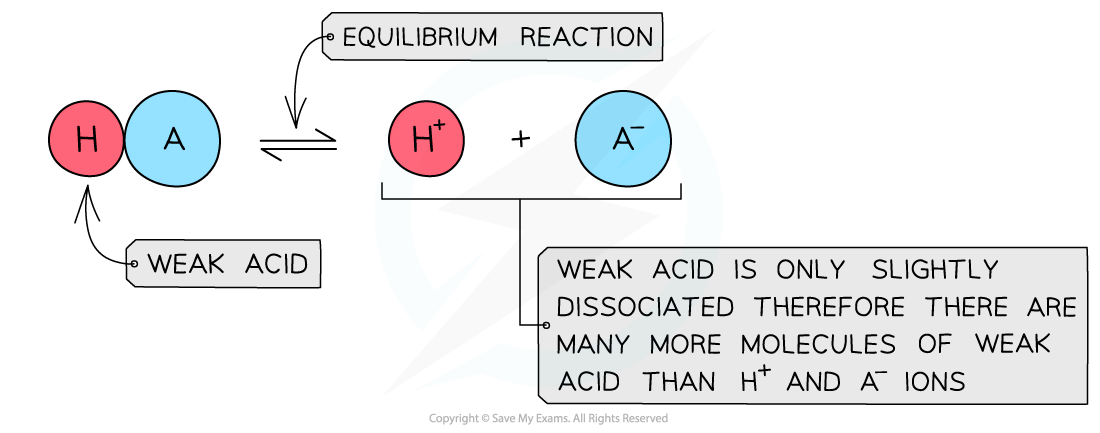# AQA A Level Chemistry复习笔记5.6.1 Acid Dissociation Constant

### Acid Dissociation Constant

#### Weak acids

• A weak acid is an acid that partially (or incompletely) dissociates in aqueous solutions
• Eg. most organic acids (ethanoic acid), HCN (hydrocyanic acid), H2S (hydrogen sulfide) and H2CO3 (carbonic acid)

• The position of the equilibrium is more over to the left and an equilibrium is establishedThe diagram shows the partial dissociation of a weak acid in aqueous solution

• As this is an equilibrium we can write an equilibrium constant expression for the reaction• This constant is called the acid dissociation constant, Ka, and has the units mol dm-3
• Values of Ka are very small, for example for ethanoic acid Ka = 1.74 x 10-5 mol dm-3
• When writing the equilibrium expression for weak acids, the following assumptions are made:
• The concentration of hydrogen ions due to the ionisation of water is negligible

• The value of Ka indicates the extent of dissociation
• The higher the value of Ka the more dissociated the acid and the stronger it is
• The lower the value of Ka the weaker the acid

#### Worked Example

Writing Ka expressionsWrite the expression for the following acids:

1. Benzoic acid, C6H5COOH
2. Carbonic acid, H2CO3### pH of Weak Acids

#### Weak acids

• The pH of weak acids can be calculated when the following is known:
• The concentration of the acid
• The Ka value of the acid

• From the Ka expression we can see that there are three variables:• However, the equilibrium concentration of [H+] and [A-] will be the same since one molecule of HA dissociates into one of each ion
• This means you can simplify and re-arrange the expression to

Ka x [HA] = [H+]2

[H+]2 = Ka x [HA]

• Taking the square roots of each side

[H+] = √(Ka x [HA])

• Then take the negative logs

pH = -log[H+] = -log√(Ka x [HA])

#### Worked Example

pH calculations of weak acidsCalculate the pH of 0.100 mol dm-3 ethanoic acid at 298 k with a Ka value of 1.74 × 10-5 mol dm-3

Ethanoic acid is a weak acid which ionises as follows:

CH3COOH (aq) ⇌ H+ (aq) + CH3COO- (aq)

Step 1: Write down the equilibrium expression to find KaStep 2: Simplify the expression

The ratio of H+ to CH3COO- ions is 1:1

The concentration of H+ and CH3COO- ions are therefore the same

The expression can be simplified to:Step 3: Rearrange the expression to find [H+]Step 4: Substitute the values into the expression to find [H+]= 1.32 x 10-3 mol dm-3

Step 5: Find the pH

pH = -log[H+]

= -log(1.32 x 10-3)

= 2.88